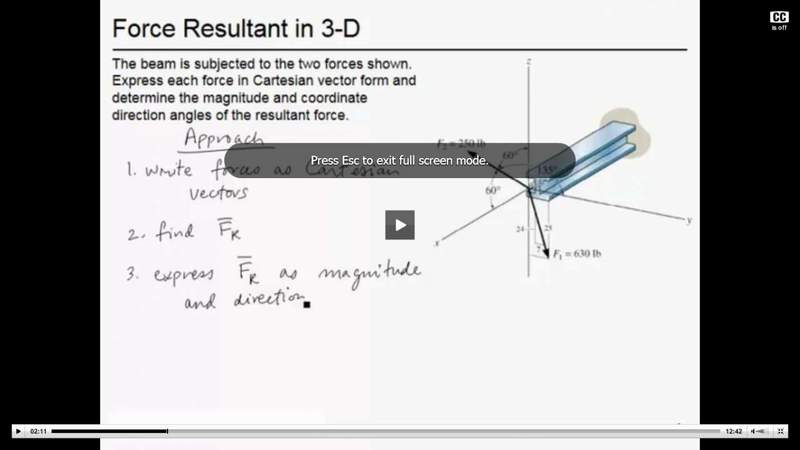# How to relate force to the z-axis

## Homework Statement

Im having trouble understanding parts about the z-axis for my statics class. You know how Fx=FcosΘ and Fy=FsinΘ. How do you express force in the z direction? And also if I have a right triangle with a leg of 24 units and a leg of 7 units and a hypotenuse of 25. I see they can skip using cosΘ and sinΘ in the force equations and can just use for example Fy=F(7/25) instead of using sin. Which legs would you choose for z-axis?

I attached an image and I am not sure i it showed up. And you can see I am trying resolve the F1 force in Cartesian vector form They have F1=630((7/25)j-(24/25)k). So I am wondering how they choose those legs for the z-axis force?shownn above

## The Attempt at a Solution

none so far

F1 is in the y-z plane. The 7-24-25 triangle attached to the vector arrow shows how the components fall out.

For F2, the angles which the vector make with each of the coordinate axes are shown.